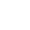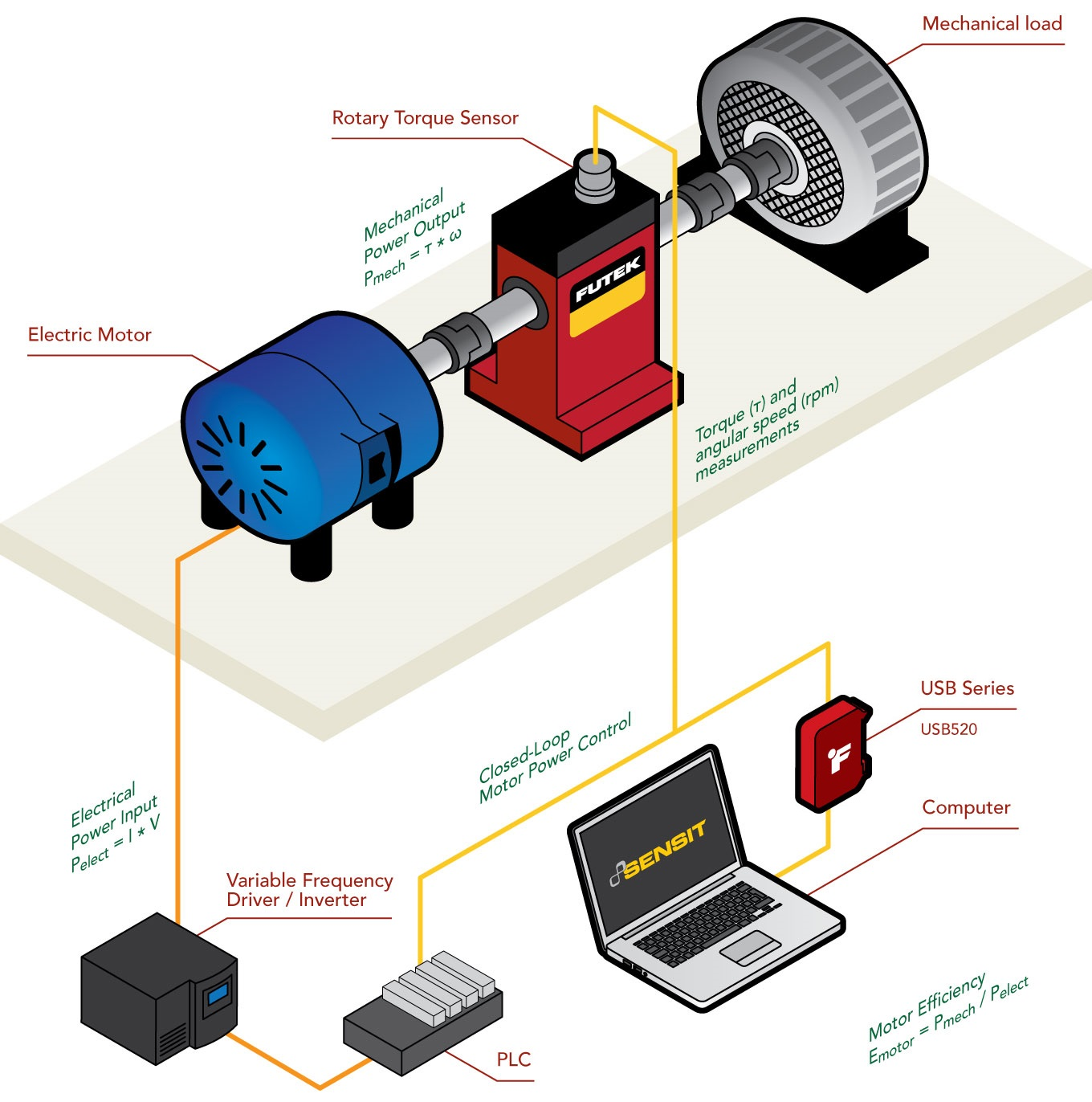Office Closure: Dec 25, 2023, to Jan 1, 2024. Order by Dec 15 for 2023 shipping. Happy holidays from FUTEK!

Applications keyboard_arrow_right   |  Electric Motor Efficiency

# Electric Motor Efficiency

Basic concepts of electric motor efficiency
Electric motors are electromechanical machines that convert electric energy into mechanical energy through a spinning shaft. Despite differences in size and type, most electric motors work based on the same basic principle: an electric current flowing through a wire coil in a magnetic field (stator) creates a force that rotates the coil (rotor), thus creating torque (spinning shaft).

In that scenario, the motor efficiency is a ratio of how well the motor converts electrical energy into mechanical energy and is calculated by dividing the electrical power fed into the motor by the mechanical power generated from the motor. Mechanical power output is then calculated based on the torque and angular speed, and electrical power input is calculated based on voltage and current supplied to the motor. Mechanical power output is always lower than the electrical power input, as energy is lost during the conversion process in various forms, such as heat, friction, ohmic losses, and noise.

Electric motor output power

As described above, electrical motors transform electrical energy into mechanical work. Two important variables determine the mechanical capabilities of a motor: angular speed and torque. Mechanical power output of the motor could be calculated by using the following formula:

Pmech = Pout = τ * ω

The consumed electrical power of the motor is defined by the following equation:

Pelet = Pin = I * V

The efficiency of the motor is calculated as mechanical output power divided by electrical input power:

Motor Efficiency = Pmech / Pelet = Pout / Pin

If the motor has 100% efficiency all electrical power is converted to mechanical energy (Pin = Pout). However such motors do not exist. Even precision-made small industrial motors have a maximum efficiency of 50-60%.

How do you measure the power output of a motor?
As detailed above, determining the power output of a motor requires measuring two variables simultaneously: torque and angular speed. For precise and accurate torque and angular speed measurement, a Rotary Torque Sensor with encoder paired with USB520 Universal Signal Conditioner is the recommended method. See the USB520 product brochure here for more information.

How it Works
1. FUTEK's Rotary Torque Sensors with Encoders paired with USB520 is an effective tool for motor power testing / auditing, feedback control as well as monitoring torque and analyzing electric motor efficiency.

2. As the motor rotates, the sensor measures the torque produced by the motor in response to the load generated by the mechanical load.

3. FUTEK has developed torque sensors with built-in encoders. These encoders measure the angle/speed (RPM) produced during this test.

4. The USB520 is the ideal signal conditioner solution for systems that require measurement of rotary torque and encoder readings, such as angle and speed. Its compact and robust design also makes the USB520 fitting for this type of industrial application.

5. Applying FUTEK's SENSIT™ Test and Measurement Software to this test platform will allow the operator to live graph and data log the results.

6. The USB520 output can be integrated with a PLC / VFD solution for closed loop motor power control.

Products in Use

Rotary Torque Sensor with Encoder paired with USB520 Universal Signal Conditioner.

All FUTEK application illustrations are strictly conceptual.

Basic concepts of electric motor efficiency
Electric motors are electromechanical machines that convert electric energy into mechanical energy through a spinning shaft. Despite differences in size and type, most electric motors work based on the same basic principle: an electric current flowing through a wire coil in a magnetic field (stator) creates a force that rotates the coil (rotor), thus creating torque (spinning shaft).

In that scenario, the motor efficiency is a ratio of how well the motor converts electrical energy into mechanical energy and is calculated by dividing the electrical power fed into the motor by the mechanical power generated from the motor. Mechanical power output is then calculated based on the torque and angular speed, and electrical power input is calculated based on voltage and current supplied to the motor. Mechanical power output is always lower than the electrical power input, as energy is lost during the conversion process in various forms, such as heat, friction, ohmic losses, and noise.

Electric motor output power

As described above, electrical motors transform electrical energy into mechanical work. Two important variables determine the mechanical capabilities of a motor: angular speed and torque. Mechanical power output of the motor could be calculated by using the following formula:

Pmech = Pout = τ * ω

The consumed electrical power of the motor is defined by the following equation:

Pelet = Pin = I * V

The efficiency of the motor is calculated as mechanical output power divided by electrical input power:

Motor Efficiency = Pmech / Pelet = Pout / Pin

If the motor has 100% efficiency all electrical power is converted to mechanical energy (Pin = Pout). However such motors do not exist. Even precision-made small industrial motors have a maximum efficiency of 50-60%.

How do you measure the power output of a motor?
As detailed above, determining the power output of a motor requires measuring two variables simultaneously: torque and angular speed. For precise and accurate torque and angular speed measurement, a Rotary Torque Sensor with encoder paired with USB520 Universal Signal Conditioner is the recommended method. See the USB520 product brochure here for more information.ApplicationsElectric-motor-efficiency
All other trademarks, service marks and logos used in this website are the property of their respective owners.applicationsElectric-Motor-Efficiency